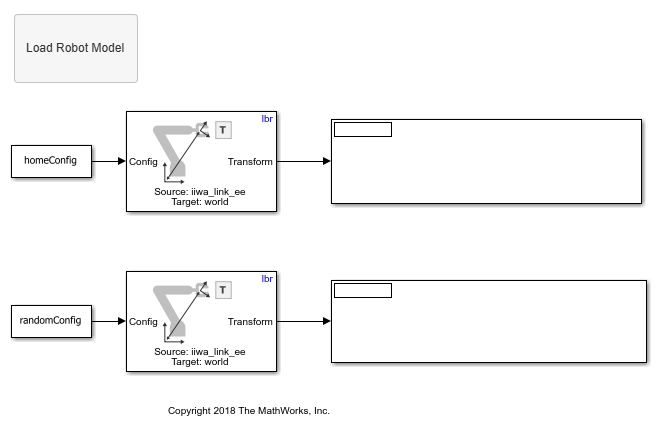# Get Transformations for Manipulator Bodies in Simulink

This example shows how to get the transformation between bodies in a `rigidBodyTree` robot model. In this example, you define a robot model and robot configuration in MATLAB® and pass them to Simulink® to be used with the manipulator algorithm block.

Load the robot model of the KUKA LBR robot as a `RigidBodyTree` object. Use the `homeConfiguration` function to get the home configuration as joint positions of the robot.

```load('exampleLBR.mat','lbr') lbr.DataFormat = 'column'; homeConfig = homeConfiguration(lbr); randomConfig = randomConfiguration(lbr); ```

Open the model. If necessary, use the Load Robot Model callback button to reload the robot model and configuration vectors.

The Get Transform block calculates the transformation from the source body to the target body. This transformation converts coordinates from the source body frame to the given target body frame. This example gives you transformations to convert coordinates from the `'iiwa_link_ee'` end effector into the `'world'` base coordinates.

```open_system('get_transform_example.slx') ```Run the model to get the transformations.